Courses

# NCERT Solution - Sound, Class 9,Science Class 9 Notes | EduRev

## Class 9 : NCERT Solution - Sound, Class 9,Science Class 9 Notes | EduRev

The document NCERT Solution - Sound, Class 9,Science Class 9 Notes | EduRev is a part of Class 9 category.
All you need of Class 9 at this link: Class 9

Chapter : Sound

1. How does the sound produced by a vibrating object in a medium reach your ear ?

OR

Explain how sound is produced by your school bell.

Ans. Air is the commonest material through which sound propagates. When a vibrating object, like prongs of tuning fork move forward, they push the molecules of the air infront of them. This is turn compresses the air, thus creating a region of high pressure and high density called compression. This compression in the air travels forward. When the prongs of the tuning fork move backward, they create a region of low pressure in the air, commonly called rarefaction. This region has low pressure low density and more volume. As the tuning fork continues to vibrate, the regions of compression in the air alternate with the regions of rarefaction. These regions alternate at the same place. The energy of vibrating tuning fork travels outward. This energy which reaches the ears, makes the ear drums to vibrate and thus we hear sound.

2. A sonar device on a submarine sends out a signal and receives an echo 5 s later. Calculate the speed of sound in water if the distance of the object from the submarine is 3625 m.

Ans. Speed of sound =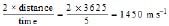3. Why are sound waves called mechanical waves ?

Ans. Some mechanical energy is required to make an object vibrate. Sound energy cannot be produced on its own. the mechanical energy of vibrating object travels through a medium and finally reaches the ear. Therefore, the sound waves are called mechanical waves.

4. Suppose you and your friend are on the moon. Will you be able to hear any sound produced by your friend?

Ans. No, I will not be able to hear sound, because Moon has no atmosphere. Therefore, no sound waves can travel to your ears and therefore, no sound is heard.

5. Which wave property determines

(a) loudness, (b) pitch ?

Ans. (a) The amplitude of the wave determines the loudness; more the amplitude of a wave, more is-the loudness produced.

(b) The pitch is determined by the frequency of the wave. Higher the frequency of a wave, more is its pitch and shriller is the sound.

6. Guess which sound has a higher pitch; guitar or car horn ?

Ans. Car horn has a higher pitch than a guitar, because sound produced by the former is shriller than the later.

7. What are wavelength, frequency, time period and amplitude of a sound wave ?

Ans. Wavelength : It is the linear distance between two consecutive compressions or two consecutive rarefactions.

Frequency : The number of compressions or rarefactions taken together passing through a point in-one second is called frequency.

Time Period : It is the time taken by two consecutive compressions or rarefactions to cross a point.

Amplitude : It is the magnitude of maximum displacement of a vibrating particle about its mean position.

8. How are the wavelength and frequency of a sound wave related to its speed ?

Ans. Speed of sound = Frequency × Wavelength

9. Calculate the wavelength of a sound wave whose frequency is 220 Hz and speed is 440 ms1 in a given medium.

Ans. Frequency = 220 Hz

Speed of sound 440 ms1

We know speed of sound = Frequency × wavelength

Þ Wavelength = = 2m

10. A person is listening to a tone of 500 Hz sitting at a distance of 450 m from the source of the sound. What is the time interval between successive compressions from the source ?

Ans. Time interval =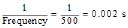11. Distinguish between loudness and intensity of sound.

Ans. The loudness depends on energy per unit area of the wave and on the response of the ear but intensity depends only on the energy per unit area of the wave and is independent of the response of the ear.

12. In which of the three media, air, water or iron, does sound travel the fastest at a particular temperature?

Ans. Sound travels fastest in iron as compared to water and air.

13. An echo is returned in 3s. What is the distance of the reflecting surface from the source, given that the speed of sound is 342 ms1 ?

Ans. Distance of reflecting body from the source of sound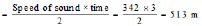14. Why are the ceiling of concert halls curved ?

Ans. The ceilings of concert halls are curved because sound after reflection from it reaches all the corners of the hall and is audible to each person in the hall.

15. What is the audible range of the average human ear ?

Ans. An average human ear can hear sound waves between frequencies 20 Hz to 20,000 Hz.

16. What is the range of frequencies associated with

(a) Infrasound ? (b) Ultrasound ?

Ans. (a) Infrasound : Sound waves between the frequencies 1 to 20-Hz

(b) Ultrasound : Sound waves of the frequencies above 20,000 Hz.

17. A submarine emits a sonar pulse, which returns from an underwater cliff in 1.02 s. If the speed of, sound in salt water is 1531 ms1, how far away is the cliff ?

Ans. Distance of cliff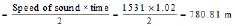18. What is sound and how is it produced ?

Ans. Sound is mechanical energy which produces sensation of hearing. When an object is set into vibrations, sound is produced.

20. Give an experiment to show that sound needs a material medium for its Propagation.

Ans. Take an electric circuit which consists of a cell, a switch and an electric bell arranged inside a bell jar, which stands on the platform of an evacuating pump.

The switch of the bell is pressed to close the electric circuit. When there is air within the bell jar, sound is heard. Air is now pumped out of the bell jar. When the air is completely removed from the bell jar, no sound is heard as it is obvious from fig. because the medium of air which has to carry energy from the bell to the bell jar, is removed. It shows that sound needs material medium for its propagation.

21. Why is sound wave called a longitudinal wave ?

Ans. Sound wave is called longitudinal wave because the particles of the medium vibrate in the direction of the propagation of wave.

22. Which characteristic of the sound helps you to identify your friend by his voice while sitting with Miens in a dark room?

Ans. The characteristic of sound is quality or timbre.

23. Flash and thunder are produced simultaneously. But thunder is heard a few seconds after the flash is seen, why ?

Ans. The speed of light is 3 × 10ms1 and the speed of sound is 344 ms1 in air. Thus, flash of lightning is seen at first, but sound takes few seconds to reach the ears.

24. A person has a hearing range from 20 Hz to 20 kHz. what are the typical wavelengths of sound waves in air corresponding to these two frequencies ? Take the speed of sound in air as 344 ms1.

Ans. Wavelength of sound of frequency 20 Hz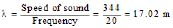wavelength of sound of frequency 20,000 Hz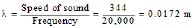25. Two children are at opposite ends of an aluminium rod. One strikes the end of the rod with a stone. Find the ratio of times taken by the sound wave in air and in aluminium to reach the second child. Given velocity of sound in air and aluminium are 346 ms1 and 6420 ms1 respectively.

Ans.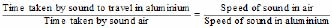Time taken by sound in air Speed of sound in aluminium

Þ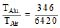Þ TAIu : TAir = 346 : 6420 = 1 : 18.55

26. The frequency of a source of sound is 100 Hz. How many times does it vibrate in a minute ?

Ans. No. of vibrations produced in 1 s = 100 Þ No. of vibration produced in 60 (sec)

(1 min) = 100 × 60 = 6000

27. Does sound follow the same laws of reflection as light does ? Explain.

Ans. Yes, sound and light follow the same laws of reflection given below :

(a) Angle of incidence at the point of incidence = Angle of reflection.

(b) At the point of incidence the incident sound wave, the normal and the reflected sound wave lie in the
same plane.

28. When a sound is reflected from a distant object, an echo is produced. Let the distance between the reflecting surface and the source of sound production remain the same. Do you hear echo sound on a hotter day ?

Ans. If the temperature rises the speed of sound will increase. This is turn will increase the minimum distance required for hearing an echo. No echo is heard because, the distance between the source of sound and reflecting body does not increase.

29. Give two practical applications of reflection of sound waves.

Ans. (i) Megaphones are designed to send sound waves in particular direction are based on the reflection of sound. (ii) In stethoscope the sound of patient's heartbeat reaches the doctor's ears by multiple reflection in the tubes.

30. A stone is dropped from the top of a tower 500 m high into a pond of water at the base of the tower. When is the splash heard at the top ? Given, g = 10 ms2 and speed of sound = 340 ms1.

Ans. For the downward journey of stone.

Initial velocity (u) = 0

Distance i.e., (height) of tower (S) = 500 m

Time of fall (t1) = ?

Acceleration due to gravity (g) = 10 ms2

We know ; S = ut gt2

500 = 0 × t1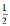× 10 × t12

Þ 500 = 5t12

Þ t12 = 100

t1 = 10 s

For the sound traveling upward

Time taken (t2)

For the sound travelling upward

Time taken (t2) =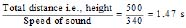Time required to hear splash = t1  t2 = 10 1.47 = 11.47 s

31. A sound wave travels at a speed of 339 ms1. If its wavelength is 1.5 cm, what is the frequency of the wave? Will it be audible ?

Ans. Speed of sound wave = 339 ms1

Wavelength of sound wave = 1.5 cm = 0.015 m

Frequency of sound wave =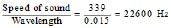The sound will not be audible, because human beings can hear only upto 20,000 Hz.

32. What is reverberation? How can it be reduced ?

Ans. Reverberation is the repeated multiple reflections of sound in any big enclosed space. It can be reduced by covering the ceiling and walls of the enclosed space with some absorbing materials like fibre board, loose woollens etc.

33. What is loudness of sound ? What factors does it depend on ?

Ans. Loudness of sound is the effect produced in the brain by the sound of different frequencies. The loudness of the sound depends on the distance of the observer from the source of sound ; lesser the distance louder the sound. It increases with the increase in amplitude and the area of the vibrating body.

34. Explain how bats use ultrasound to catch their prey.

Ans. The bats produce high pitched ultrasonic waves which are not heard by human beings. The ultrasonic waves on striking the insect send back an echo, which is heard by the bat. As the echo is heard by the bat it hoves on the insect and catches it.

35. How is ultrasound used for cleaning ?

Ans. The object to be cleaned is put in a tank fitted with ultrasonic vibrator. The tank is filled with water containing detergent. As the ultrasonic vibrator is switched on the detergent rub against the object at a very high speed and hence clean it.

36. Explain the working and application of a sonar.

Ans. SONAR is a device for determining water depth and locating underwater objects like reefs, submarines and schools of fish.

On striking the bottom of the ocean, the ultrasonic wave is reflected upward toward the ship. This wave is received by a suitable receiver. The time of travel from the source of sound to the receiver is noted. We can calculate the depth of ocean floor if the velocity of sound in water is known

Depth of ocean floor =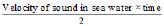For Example : If it takes 2.4 s to record echo by the sonar

Velocity of sound in sea water = 1450 ms1

Depth of ocean floor =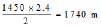Offer running on EduRev: Apply code STAYHOME200 to get INR 200 off on our premium plan EduRev Infinity!

,

,

,

,

,

,

,

,

,

,

,

,

,

,

,

,

,

,

,

,

,

,

,

,

,

,

,

;